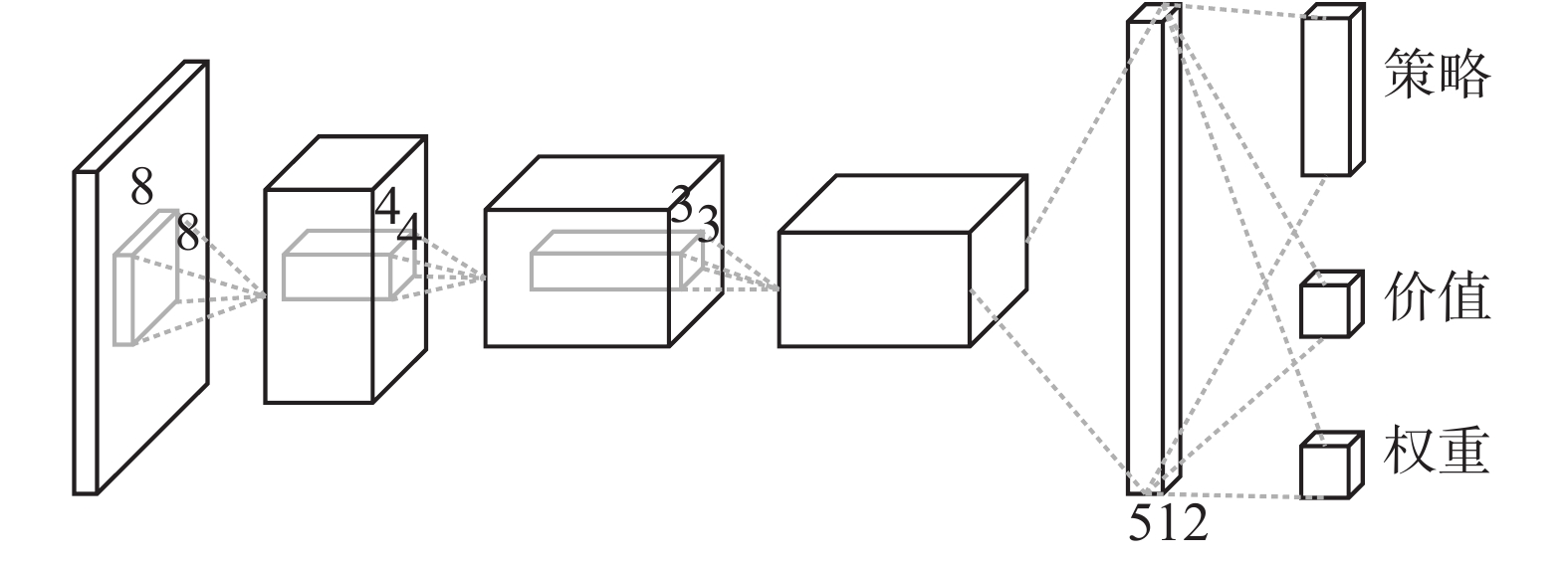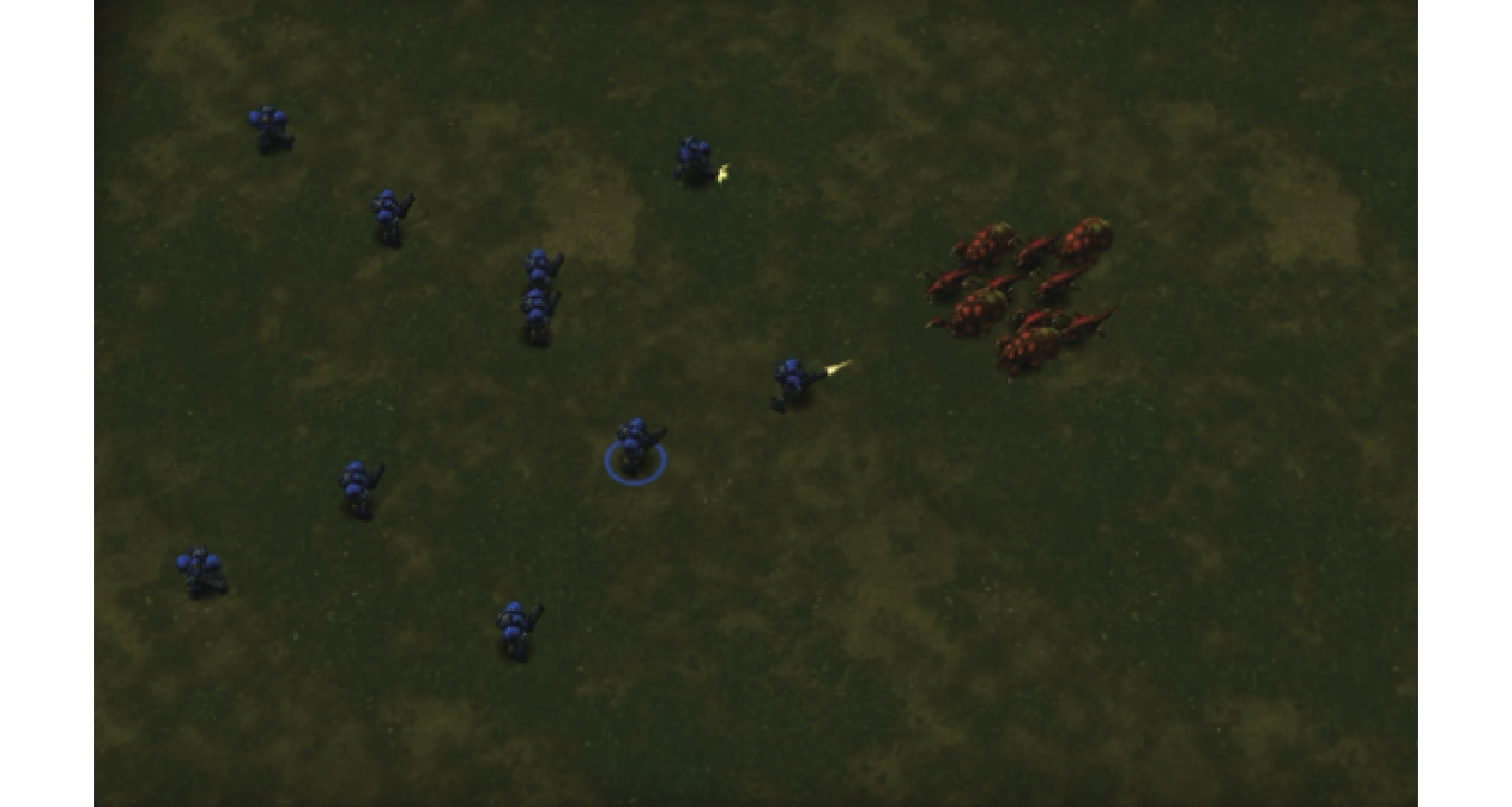﻿ 深度强化学习中状态注意力机制的研究
«上一篇文章快速检索 高级检索

 智能系统学报2020, Vol. 15Issue (2): 317-322  DOI: 10.11992/tis.2018090330

### 引用本文SHEN Xiangxiang, HOU Xinwen, YIN Chuanhuan. State attention in deep reinforcement learning[J]. CAAI Transactions on Intelligent Systems, 2020, 15(2): 317-322. DOI: 10.11992/tis.201809033.### 文章历史

1. 北京交通大学 交通数据分析与挖掘北京市重点实验室，北京 100044;
2. 中国科学院自动化研究所 智能系统与工程研究中心，北京 110016

State attention in deep reinforcement learning
SHEN Xiangxiang 1, HOU Xinwen 2, YIN Chuanhuan 11. Beijing Key Laboratory of Traffic Data Analysis and Mining, Beijing Jiaotong University, Beijing 100044, China;
2. Center for Research on Intelligent System and Engineering, Institute of Automation, Chinese Academy of Sciences, Beijing 110016, China
Abstract: Through artificial intelligence, significant achievements beyond the human level have been made in the field of board games and video games since the emergence of deep reinforcement learning. However, the real-time strategic game StarCraft is a huge challenging platform for artificial intelligence researchers due to its huge state space and action space. Considering that the level of baseline agents trained by DeepMind using classical deep reinforcement learning algorithm A3C in StarCraft II mini-game is still far from that of ordinary amateur players, by adopting a more simplified network structure and combining the attention mechanism with rewards in reinforcement learning, an A3C algorithm based on state attention is proposed to solve this problem. The trained agent achieves the highest score, which is 71 points higher than Deepmind’s baseline agent in individual interplanetary mini games with fewer feature layers.
Key words: deep learning    reinforcement learning    attention mechanism    A3C    StarCraft II mini-games    agent    micromanagement

1)采用的网络结构比Deepmind提供的基线智能体的网络结构更加简洁。

2)将强化学习中的奖励与注意力机制结合起来，每一个时间步，智能体更加关注有价值的游戏状态。

1 强化学习

 ${R_t} = \sum\limits_{k = 0}^\infty {{\gamma ^k}r\left( {{s_{t + k}},{a_{t + k}}} \right)}$

 ${\max _\pi }{E_{s\sim {P_0}}}\left[ {{R_t}|{s_t} = s} \right]$

${P_0}$ 为状态 $s$ 的先验分布，动作值函数表示为

 ${Q^\pi }\left( {s,a} \right) = {E_\pi }\left[ {{R_t}|{s_t} = s,{a_t} = a} \right]$

 ${V^\pi }\left( s \right) = {E_\pi }\left[ {{R_t}|{s_t} = s} \right]$

A3C算法是将策略函数和价值函数相结合的强化学习方法，对目标函数式(1)：

 ${J_\theta }\left( s \right) = {E_{{\pi _\theta }}}\left[ {{R_t}|{s_t} = s} \right]$ (1)

 ${\nabla _\theta }{J_\theta }\left( s \right) = {E_{{\pi _\theta }}}\left[ {\nabla _\theta }\ln {\pi _\theta }\left( {{a_t}|{s_t}} \right) {R_t}|{s_t} = s \right]$

 ${\nabla _\theta }{J_\theta }\left( s \right) = {E_{{\pi _\theta }}}\left[ {\nabla _\theta }\ln {\pi _\theta }\left( {{a_t}|{s_t}} \right) \left[ {{R_t} - b\left( {{s_t}} \right)} \right]|{s_t} = s \right]$

 ${\nabla _\theta }{J_\theta }\left( s \right) = {E_{{\pi _\theta }}}\left[ \begin{array}{l} {\nabla _\theta }\ln {\pi _\theta }\left( {{a_t}|{s_t}} \right) \left[ {Q^\pi }\left( {{s_t},{a_t}} \right) - {V^\pi }\left( {{s_t}} \right) \right]|{s_t} = s \\ \end{array} \right]$

 ${\left[ {{G^\pi }\left( {{s_t}} \right) - V_{\theta '}^\pi \left( {{s_t}} \right)} \right]^2}$ (2)

 ${G^\pi }\left( {{s_t}} \right) = {E_{{\pi _\theta }}}\left[ {\sum\limits_{k = 0}^n {\gamma ^k}r\left( {{s_{t + k}},{a_{t + k}}} \right) + {\gamma ^{n + 1}}V_{\theta '}^\pi \left( {{s_{t + n + 1}}} \right) } \right]$

 ${J_\theta }\left( {{s_t}} \right) = {E_{{\pi _\theta }}}\left[ {{A^\pi }\left( {{s_t},{a_t}} \right)} \right] + \delta H\left[ {{\pi _\theta }\left( {{s_t}} \right)} \right]$

 ${\nabla _\theta }{J_\theta }\left( {{s_t}} \right) = {E_{{\pi _\theta }}}\left[ {\nabla _\theta }\ln {\pi _\theta }\left( {{a_t}|{s_t}} \right) \left[ {Q^\pi }\left( {{s_t},{a_t}} \right) - {V^\pi }\left( {{s_t}} \right) \right]{\rm{ + }} \delta {\nabla _\theta }H\left[ {{\pi _\theta }\left( {{s_t}} \right)} \right] \right]$

A3C算法同时为了提高学习的稳定性并且加快学习速度，利用异步的方法，将多个智能体在不同的线程中运行，共同更新一个策略网络。

2 基于状态注意力的A3C算法

 ${R_t} = \sum\limits_{k = 0}^\infty {{\gamma ^k}{w_\vartheta }\left( {{s_{t + k}}} \right)r\left( {{s_{t + k}},{a_{t + k}}} \right)}$

 ${Q^\pi }\left( {{s_t},{a_t}} \right) = {w_\vartheta }\left( {{s_t}} \right)r\left( {{s_t},{a_t}} \right) + \gamma {Q^\pi }\left( {{s_{t + 1}},{a_{t + 1}}} \right)$ (4)
 ${V^\pi }\left( {{s_t}} \right) = {E_{{\pi _\theta }}}\left[ {w_\vartheta }\left( {{s_t}} \right)r\left( {{s_t}} \right) + \gamma {V^\pi }\left( {{s_{t + 1}}} \right) \right]$ (5)

 ${J'_{{w_\vartheta }}}\left( {{s_t}} \right) = - {\left[ {G_{{w_\vartheta }}^\pi \left( {{s_t}} \right) - {V^\pi }\left( {{s_t}} \right)} \right]^2}$ (6)

 $G_{{w_\vartheta }}^\pi \left( {{s_t}} \right) = {E_{{\pi _\theta }}}\left[ \displaystyle\sum\limits_{k = 0}^n {\gamma ^k}{w_\vartheta }\left( {{s_{t + k}}} \right) r\left( {{s_{t + k}},{a_{t + k}}} \right) + {\gamma ^{n + 1}}{V^\pi }\left( {{s_{t + n + 1}}} \right) \right]$

 ${\nabla _\vartheta }{{J'}_{{w_\vartheta }}}\left( {{s_t}} \right) = - 2\left[ {G_{{w_\vartheta }}^\pi \left( {{s_t}} \right) - {V^\pi }\left( {{s_t}} \right)} \right] {\nabla _{{w_\vartheta }}}G_{{w_\vartheta }}^\pi \left( {{s_t}} \right){\nabla _\vartheta }{w_\vartheta }\left( {{s_t}} \right)$
3 实验验证

3.1 网络结构Download: 图 1 网络结构 Fig. 1 Network architecture
3.2 实验结果验证Download: 图 2 战胜跳虫和毒爆虫游戏界面截图 Fig. 2 The screenshot of DefeatZerglingsAndBanelings表 1 人类和智能体获取的平均分数表 Tab.1 Averaged results for human baselines and agents

4 结束语

  LI Yuxi. Deep reinforcement learning: an overview[EB/OL]. [2018-01-17]https://arxiv.org/abs/1701.07274. (0)  MNIH V, KAVUKCUOGLU K, SILVER D, et al. Human-level control through deep reinforcement learning[J]. Nature, 2015, 518(7540): 529-533. DOI:10.1038/nature14236 (0)  SILVER D, HUANG A, MADDISON C J, et al. Mastering the game of Go with deep neural networks and tree search[J]. Nature, 2016, 529(7587): 484-489. DOI:10.1038/nature16961 (0)  VINYALS O, EWALDS T, BARTUNOV S, et al. StarCraft II: a new challenge for reinforcement learning[EB/OL]. [2018-01-17]https://arXiv: 1708.04782, 2017. (0)  ONTANON S, SYNNAEVE G, URIARTE A, et al. A survey of real-time strategy game AI research and competition in StarCraft[J]. IEEE transactions on computational intelligence and AI in games, 2013, 5(4): 293-311. DOI:10.1109/TCIAIG.2013.2286295 (0)  SYNNAEVE G, BESSIERE P. A dataset for StarCraft AI & an example of armies clustering[C]//Artificial Intelligence in Adversarial Real-Time Games. Palo Alto, USA, 2012: 25–30. (0)  SYNNAEVE G, BESSIÈRE P. A Bayesian model for opening prediction in RTS games with application to StarCraft[C]//Proceedings of 2011 IEEE Conference on Computational Intelligence and Games. Seoul, South Korea, 2011: 281–288. (0)  JUSTESEN N, RISI S. Learning macromanagement in starcraft from replays using deep learning[C]//Proceedings of 2017 IEEE Conference on Computational Intelligence and Games. New York, USA, 2017: 162–169. (0)  DODGE J, PENNEY S, HILDERBRAND C, et al. How the experts do it: assessing and explaining agent behaviors in real-time strategy games[C]//Proceedings of the 2018 CHI Conference on Human Factors in Computing Systems. Montreal QC, Canada, 2018. (0)  PENNEY S, DODGE J, HILDERBRAND C, et al. Toward foraging for understanding of starcraft agents: an empirical study[C]//Proceedings of the 23rd International Conference on Intelligent User Interfaces. Tokyo, Japan, 2018: 225–237. (0)  PENG Peng, WEN Ying, YANG Yaodong, et al. Multiagent bidirectionally-coordinated nets for learning to play starcraft combat games[EB/OL]. [2018-01-17]https://arXiv: 1703.10069, 2017. (0)  SHAO Kun, ZHU Yuanheng, ZHAO Dongbin, et al. StarCraft micromanagement with reinforcement learning and curriculum transfer learning[J]. IEEE transactions on emerging topics in computational intelligence, 2019, 3(1): 73-84. DOI:10.1109/TETCI.2018.2823329 (0)  WENDER S, WATSON I. Applying reinforcement learning to small scale combat in the real-time strategy game StarCraft: Broodwar[C]//Proceedings of 2012 IEEE Conference on Computational Intelligence and Games. Granada, Spain, 2012: 402–408. (0)  DENIL M, BAZZANI L, LAROCHELLE H, et al. Learning where to attend with deep architectures for image tracking[J]. Neural computation, 2012, 24(8): 2151-2184. DOI:10.1162/NECO_a_00312 (0)  BAHDANAU D, CHO K, BENGIO Y, et al. Neural machine translation by jointly learning to align and translate[C]//Proceedings of International Conference on Learning Representations. 2015. (0)  MNIH V, HEESS N, GRAVES A, et al. Recurrent models of visual attention[C]//Proceedings of the 27th International Conference on Neural Information Processing Systems. Montreal, Canada, 2014: 2204–2212. (0)  MNIH V, BADIA A P, MIRZA M, et al. Asynchronous methods for deep reinforcement learning[C]//Proceedings of the 33rd International Conference on Machine Learning. New York USA, 2016: 1928-1937. (0)  WILLIAMS R J. Simple statistical gradient-following algorithms for connectionist reinforcement learning[J]. Machine learning, 1992, 8(3/4): 229-256. DOI:10.1023/A:1022672621406 (0)  ILYAS A, ENGSTROM L, SANTURKAR S, et al. Are deep policy gradient algorithms truly policy gradient algorithms? [EB/OL]. [2018-01-17]https://arXiv: 1811.02553, 2018. (0)  DeepMind. DeepMind mini games[EB/OL]. (2017-08-10)[2018-09-10]. https://github.com/deepmind/pysc2/blob/master/docs/mini_games.md. (0)